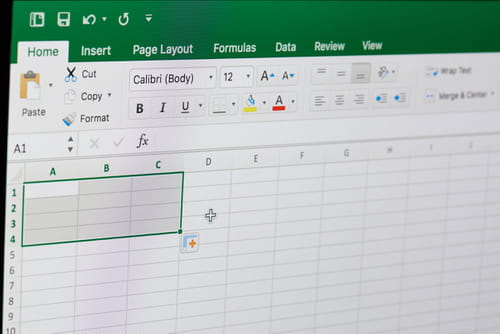2
Thanks

A few words of thanks would be greatly appreciated.

# Use a Macro to Create New Workbook and Copy Data in Excel

Often we need to carry out manipulations in Excel documents and apply them to multiple rows, columns and sheets. To do this manually takes too much time and allows for errors to creep in, so Excel offers the option of Macros, which carry out data manipulation operations for you. In this article we will show you how to use a pre-existing Macro to create a new workbook and copy data into a new spreadsheet.## Use a Macro to Create a New Workbook and Copy Data

• 1. Make a backup of the spreadsheet
• 2. Open the work book
• 3. Press ALT + F11 to open VBE
• 4. Go to Insert > Module
• 5. Copy and paste the following code into the module:
`Sub details()    Dim thisWB  As String    Dim newWB As String    thisWB = ActiveWorkbook.Name    On Error Resume Next        Sheets("tempsheet").Delete        On Error GoTo 0    Sheets.Add        ActiveSheet.Name = "tempsheet"    Sheets("Sheet1").Select    If ActiveSheet.AutoFilterMode Then            Cells.Select    On Error Resume Next    ActiveSheet.ShowAllData    On Error GoTo 0    End If    Columns("B:B").Select        Selection.Copy    Sheets("tempsheet").Select        Range("A1").Select        ActiveSheet.Paste        Application.CutCopyMode = False    If (Cells(1, 1) = "") Then            lastrow = Cells(1, 1).End(xlDown).Row    If lastrow <> Rows.Count Then                Range("A1:A" & lastrow - 1).Select                Selection.Delete Shift:=xlUp            End If    End If    Columns("A:A").Select        Columns("A:A").AdvancedFilter Action:=xlFilterCopy, _                    CopyToRange:=Range("B1"), Unique:=True    Columns("A:A").Delete    Cells.Select        Selection.Sort _                Key1:=Range("A2"), Order1:=xlAscending, _                Header:=xlYes, OrderCustom:=1, _                MatchCase:=False, Orientation:=xlTopToBottom, _                DataOption1:=xlSortNormal    lMaxSupp = Cells(Rows.Count, 1).End(xlUp).Row    For suppno = 2 To lMaxSupp    Windows(thisWB).Activate    supName = Sheets("tempsheet").Range("A" & suppno)    If supName <> "" Then    Workbooks.Add                ActiveWorkbook.SaveAs supName                newWB = ActiveWorkbook.Name    Windows(thisWB).Activate    Sheets("Sheet1").Select                Cells.Select    If ActiveSheet.AutoFilterMode = False Then                    Selection.AutoFilter                End If    Selection.AutoFilter Field:=2, Criteria1:="=" & supName, _                            Operator:=xlAnd, Criteria2:="<>"    lastrow = Cells(Rows.Count, 2).End(xlUp).Row    Rows("1:" & lastrow).Copy    Windows(newWB).Activate                ActiveSheet.Paste    ActiveWorkbook.Save                ActiveWorkbook.Close    End If    Next    Sheets("tempsheet").Delete    Sheets("Sheet1").Select        If ActiveSheet.AutoFilterMode Then            Cells.Select            ActiveSheet.ShowAllData        End If    End Sub`
• 6. Press F5 to run the macro
• 7. Check it has worked

Image © Dzmitry Kliapitski - 123rf.com

2
Thanks

A few words of thanks would be greatly appreciated.